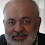## Monday, December 22, 2008

### Archimedes' Arbelos and Square 2

Dynamic Geometry Software. Step-by-Step construction, Manipulation, and animation
In the figure, a circle D is inscribed in the arbelos ABC (AB, BC and AC are semicircles), prove that KLFM is a square.gogeometry.com/geometry/archimedes_arbelo_circle_square_2.htm

1.Very interesting property! Here is my proof. The point E is the internal center of similitude of the red circle and the largest circle. Hence, KL is the image of
AC by the homothety with center E. This implies KL is parallel to AC. On the other hand, by the theorem of Pappus, the distance between D and AC is equal to the diameter of the red circle. Therefore KLFM is a square.

I would like to propose one more problem for this figure: Prove that AC is larger than or equal to 3MF.

1.It is direct from Pappus chain theorem

2.Carlos Farfàn S. excelente demostración de la propiedad. Gracias por compartir este gran programa animado geogebra